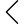627 products found
• s
• m
• l
• xl
• s
• m
• l
• xl
• s
• m
• l
• xl
• s-m
• l-xl
• s-m
• l-xl
• s
• m
• l
• xl
• s
• m
• l
• xl
• s
• m
• l
• xl
• s
• m
• l
• xl
• s
• m
• l
• xl
• s
• m
• l
• xl
• s
• m
• l
• xl
• s
• m
• l
• xl
• s
• m
• l
• xl
• s
• m
• l
• xl
• s
• m
• l
• xl
• s
• m
• l
• xl
• s
• m
• l
• xl
• s
• m
• l
• xl
• s
• m
• l
• xl
• s
• m
• l
• xl
• s
• m
• l
• xl
• s
• m
• l
• xl
• s
• m
• l
• xl
• s
• m
• l
• xl
• s
• m
• l
• xl
• s
• m
• l
• xl
• s
• m
• l
• xl
• s
• m
• l
• xl
• s
• m
• l
• xl
• s
• m
• l
• xl
• s
• m
• l
• xl
• s
• m
• l
• xl
• s
• m
• l
• xl
• s
• m
• l
• xl
• s
• m
• l
• xl
• 2xl
• s
• m
• l
• xl
LPU CMU Tanktop
\$169.00
• s
• m
• l
• xl
LPU CMU Tanktop
\$139.00
• s
• m
• l
• xl
LPU CMU Tanktop
\$169.00
• s
• m
• l
• xl
LPU CMU Tanktop
\$169.00
•• 1
• 2
• 3
• 16